# Gauss Law MCQ Level - 2

## 25 Questions MCQ Test Exclusive Video Lectures of Class 12 Physics by Experts | Gauss Law MCQ Level - 2

Description
This mock test of Gauss Law MCQ Level - 2 for Class 12 helps you for every Class 12 entrance exam. This contains 25 Multiple Choice Questions for Class 12 Gauss Law MCQ Level - 2 (mcq) to study with solutions a complete question bank. The solved questions answers in this Gauss Law MCQ Level - 2 quiz give you a good mix of easy questions and tough questions. Class 12 students definitely take this Gauss Law MCQ Level - 2 exercise for a better result in the exam. You can find other Gauss Law MCQ Level - 2 extra questions, long questions & short questions for Class 12 on EduRev as well by searching above.
QUESTION: 1

### In a region with a uniform electric field, the number of lines of force per unit area is E. If a spherical metallic condcutor is placed in the area, the field inside the conductor will be

Solution:

There is no field inside a metallic conductor  E = 0

QUESTION: 2

### If one penetrates a uniformly charged spherical cloud electric field strength

Solution:

In side the sphere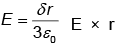therefore field decreases directly as the distance form the centre.

QUESTION: 3

### An unchanged metal sphere is placed between two equal and oppositely charged metal plates. The nature of lines of force will be

Solution:

Electric field lines always normal to surface as surface is always equipotential. So option b.

QUESTION: 4

Consider two concertric spherical surfaces S1 with radius a and S2 with radius 2a, both centred on the origin. There is a charge +q at the origin and no other c harges, Compare the flux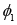through S1 with the flux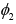through S2 .

Solution: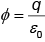as charge is same in both total flux will be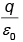for both of them.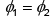QUESTION: 5

Under what conditions can the electric flux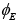be found through a closed surface?

Solution:

For total flux we need to know the total charge enclosed inside so option 'b' is correct.

QUESTION: 6

Three charges of q1 = 1 × 10-6 C, q2 = 2 × 10-6 C and q3 = -3 ×10-6 C have been placed as shown, Then the net electric flux will be maximum for the surface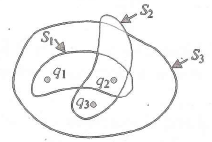Solution: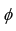will be max if charge enclosed is max S1 has max charge so flux will be max from S1.

QUESTION: 7

In a region of space, the electric field is given by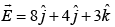. The electric flux through a surface or area of 100 units in x - y plane is

Solution:

Only field lines normal to surface will cross, so only 2 component will cross 3 ×100 = 300 units.

QUESTION: 8

A flat square surface with sides of length L is described by the equations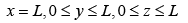The electric flux through the square due to a positive point charge q located at the origin (x = 0, y = 0, z = 0 ) is

Solution:

As charge is at corner total flux will be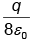, but flux from one face will be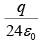.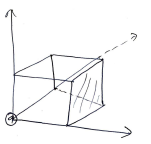QUESTION: 9

Two conducting plates X and Y, each having large surface area A (on one side) are placed parallel to each other. The plate X is given a charge Q whereas the other is neutral. The electric field at a point in between the plates is given by :

Solution: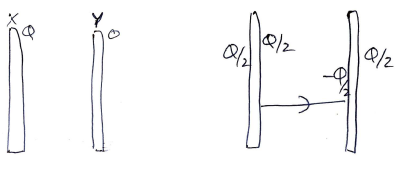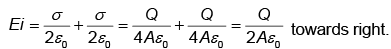QUESTION: 10

Law stated as flux is 1/Eo times the total charge is

Solution:
QUESTION: 11

A hemispherical surface of radius R is placed with its cross-section perpendicular to a uniform elcetric field E as shown in Fig. Flux linked with its curved surface is :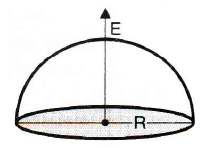Solution:

As total flux is zero.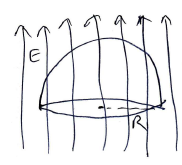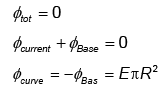QUESTION: 12

The Fig. shows two parallel equipotential surfaces A and B at same potential kept at a distance r apart from each other. A point cahrge -q is taken from surface A to B, the amount of net work done W will be :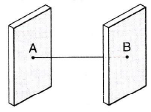Solution:

As surfaces are equipotential
ΔV = 0
W = qΔV = 0

*Multiple options can be correct
QUESTION: 13

An ellipsoidal cavity is curved within a perfect conductor. A positive charge q is placed at the centre of the cavity. The points A and B are on the cavity surface as shown in Fig.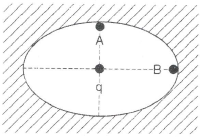Solution:

Potential will be same at A and B but as field is stranger as sharper edges so B will have stranger field as its radius is smaller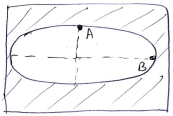QUESTION: 14

Two thin infinite sheets have uniform surface densities of charge +σ and - σ . Electric field in the space between the two sheets is :

Solution: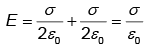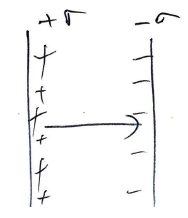QUESTION: 15

Fig. shows a hemisphere of radius 'R'. A point charge q is kept at distance 'y' above the centre such that y → 0.
Electric flux through the curved surface is :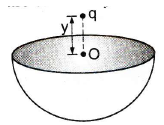Solution:

As charge may be considered to be at face center, q/2 will be inside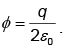QUESTION: 16

A and B are two concentric spheres, If A is given a charge Q while B is earthed as shown in fig, then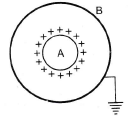Solution:

Outward charge will flow so field will only be in between A and B outside B or inside A, net charge is zero So E = 0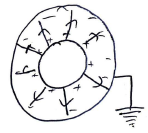QUESTION: 17

A solid metallic sphere has a charge +3Q concentric with this sphere is a conducting spherical shell having charge -Q. The radius of the sphere is a and that of the spherical shell is b(>a). What is the electric field at a distance r (a <r <b) from the centre?

Solution:

We don't have to consider charges outside and as point is outside the solid sphere, charges can be considered to be at cneter So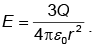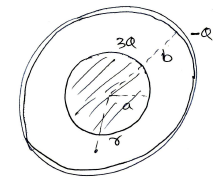QUESTION: 18

There are two concentric metal shells of radii r1 and r2 (<r1). If the outer shell has a charge q and the inner shell is grounded, the charge on the inner shell is :

Solution: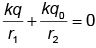as inner shell is grounded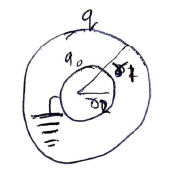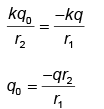QUESTION: 19

A solid conducting sphere having a charge Q is surrounded by an uncharged concentric conducting hollow spherical shell. Let the potential difference between the surface of the solid sphere and that of the outer surface of the hollow shell be V. If the shell is now given a charge -3Q, the new potential difference between the same two surface is :

Solution: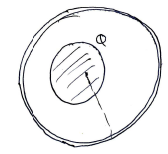If extra charge is given to outer shell there is no charge is P.Δ (discussed in class.)

QUESTION: 20

A uniformly charged an infinitely long line having a  iinear charge density 'λ' is placed at a normal distance y from a point O, Consider s sphere of radius R with O as centre and R > y. Electric flux through the surface of the sphere is :

Solution:

Part inside =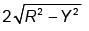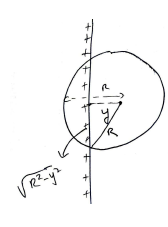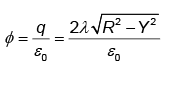QUESTION: 21

An insulating solid sphere of radius 'R' is charged in a non-uniform manner such that volume charge density P(A/r), where A is a positive constant and r the distance from centre. Electric field strength at any inside point at distance r1 is :

Solution: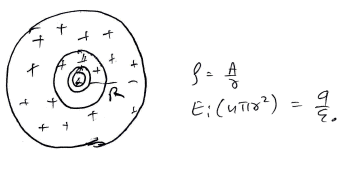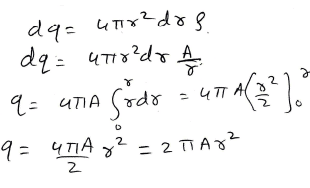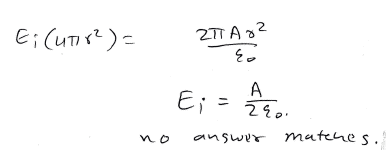QUESTION: 22

Three infinitely long charge sheets are placed as shown in figure. The electric field at point P is :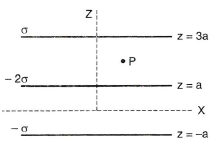Solution: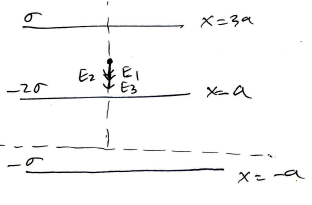All the fields will be directed downwards.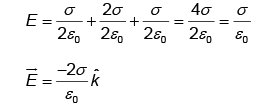QUESTION: 23

A spherical portion has been removed from a solid sphere having a charge distributed uniformly in its volume as shown in the fig. The electric field inside the emptied space is :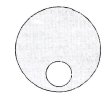Solution:

E inside is cont (class question)

QUESTION: 24

Figure shows an imaginary cube of edge L/2. An uniformly charged rod of length L moves towards left at a small but constant speed v. At t = 0, the left end just touches the centre of the face of the cube opposite it. Which of the graphs shown in the figure represents the flux of the electric field through the cube as the rod goes through it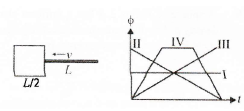Solution:

Class question.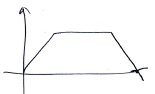QUESTION: 25

A conducting sphere S1 intersects a closed surfae S2 as shown in the figure. A positive charge q is placed at a point P. What is the value of electric flux through the surface S2?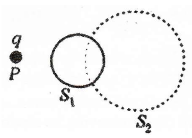Solution: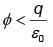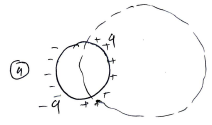As we don't know how much charge will be enclosed.